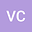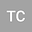Existence of exponential attractor to $p(x)$-laplacian via the $l$-trajectories method.
••Thays Couto
Author Profile## Abstract

This article is devoted to the study of the existence of an exponential attractor for a family of problems, in which diffusion $d_{\lambda}$ blows up in localized regions inside the domain \begin{equation*} \begin{cases} u_t^\lambda-\mathrm{div}(d_\lambda(x)(|\nabla u^\lambda|^{p(x)-2}+\eta ) \nabla u^\lambda)+ |u^\lambda|^{p(x)-2}u^\lambda=B(u^\lambda), & \mbox{ in } \Omega \\ u^\lambda = 0, & \mbox{ on } \partial\Omega\\ u^\lambda(0)=u^\lambda_0 \in L^2(\Omega),& \end{cases} \end{equation*} and their limit problem via the $l$-trajectory method.
06 Jun 2022Submitted to Mathematical Methods in the Applied Sciences
07 Jun 2022Submission Checks Completed
07 Jun 2022Assigned to Editor
24 Sep 2022Reviewer(s) Assigned
24 Sep 2022Review(s) Completed, Editorial Evaluation Pending
24 Sep 2022Editorial Decision: Revise Major